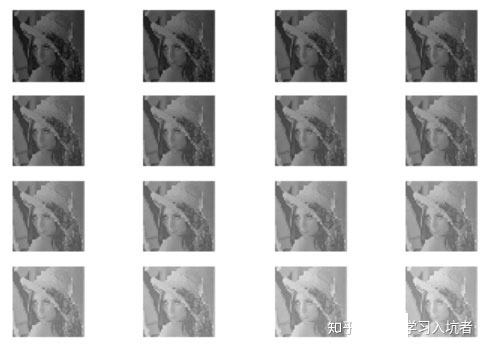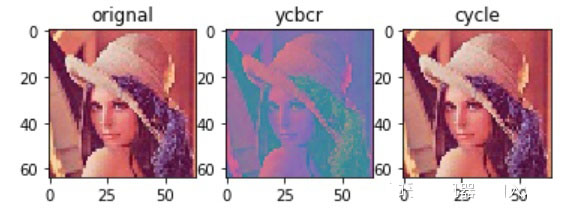﻿ python实现RGB与YCBCR颜色空间转换_python_脚本之家
python# python实现RGB与YCBCR颜色空间转换

## 1、灰度值和亮度的关系```import numpy as np
import matplotlib.pyplot as plt
import imageio
# 设置每次循环像素的增加量
shift = 6*np.ones(shape=(64, 64))
plt.figure()

for i in range(1, 17):
plt.subplot(4, 4, i)
plt.imshow(image/255, cmap="gray", vmin=0, vmax=1)
plt.axis("off")
image = image + shift```

## 2、RGB颜色空间与颜色控制

RGB模型在硬件设备中被广泛的使用，通过R（红色）、G（绿色）、B（蓝色）三者进行叠加可以形成更多的颜色。RGB颜色空间和后面将要进行介绍的YCbCr颜色空间和HSV颜色空间存在线性的变换关系，所以只要拥有RGB图像就能得到其它颜色空间的图像。• （1）创建一幅3通道的空图像
• （2）给3通道空图像的R通道添加一幅单通道图像
• （3）给3通道图像的R通道像素值不断增加偏移量
```# 1：创建一幅3通道的空图像
= np.zeros(shape=(64, 64, 3))
# 2：给3通道空图像的R通道添加一幅单通道图像
image[:, :, 0] = image[:, :, 0] + r
shift = 4*np.ones(shape=(64, 64))
plt.figure()

for i in range(1, 17):
plt.subplot(4, 4, i)
plt.imshow(image/255, vmin=0, vmax=1)
plt.axis("off")
# （3）给3通道图像的R通道像素值不断增加偏移量
image[:, :, 0] = image[:, :, 0] + shift```

## 3、YCbCr颜色空间及与RGB的变换关系

YCbCr颜色空间中的Y是亮度通道，Cb是蓝色分量，Cr是红色分量。它在电视系统中比较常用，比如早期的黑白电视机使用彩色电视信号线，就可以单独使用亮度值；这种功能RGB颜色空间就做不到，因为我们不能仅仅使用RGB中某个通道作为亮度信号来使用。

YCbCr和RGB存在线性的变换关系，本文介绍的变换矩阵来自ITU.BT-601，所规定的变换矩阵Trans形式如下：```def rgb2ycbcr(rgb_image):
"""convert rgb into ycbcr"""
if len(rgb_image.shape)!=3 or rgb_image.shape!=3:
raise ValueError("input image is not a rgb image")
rgb_image = rgb_image.astype(np.float32)
# 1：创建变换矩阵，和偏移量
transform_matrix = np.array([[0.257, 0.564, 0.098],
[-0.148, -0.291, 0.439],
[0.439, -0.368, -0.071]])
shift_matrix = np.array([16, 128, 128])
ycbcr_image = np.zeros(shape=rgb_image.shape)
w, h, _ = rgb_image.shape
# 2：遍历每个像素点的三个通道进行变换
for i in range(w):
for j in range(h):
ycbcr_image[i, j, :] = np.dot(transform_matrix, rgb_image[i, j, :]) + shift_matrix
return ycbcr_image```

```def ycbcr2rgb(ycbcr_image):
"""convert ycbcr into rgb"""
if len(ycbcr_image.shape)!=3 or ycbcr_image.shape!=3:
raise ValueError("input image is not a rgb image")
ycbcr_image = ycbcr_image.astype(np.float32)
transform_matrix = np.array([[0.257, 0.564, 0.098],
[-0.148, -0.291, 0.439],
[0.439, -0.368, -0.071]])
transform_matrix_inv = np.linalg.inv(transform_matrix)
shift_matrix = np.array([16, 128, 128])
rgb_image = np.zeros(shape=ycbcr_image.shape)
w, h, _ = ycbcr_image.shape
for i in range(w):
for j in range(h):
rgb_image[i, j, :] = np.dot(transform_matrix_inv, ycbcr_image[i, j, :]) - np.dot(transform_matrix_inv, shift_matrix)
return rgb_image.astype(np.uint8)```

```import numpy as np
import imageio
import matplotlib.pyplot as plt
ycbcr_image = rgb2ycbcr(rgb_image)
cycle_image = ycbcr2rgb(ycbcr_image)
images = [rgb_image, ycbcr_image, cycle_image]
titles = ["orignal", "ycbcr", "cycle"]
for i in range(1, len(images)+1):
plt.subplot(1, 3, i)
plt.title(titles[i-1])
plt.imshow(images[i-1]/255)``````import cv2
ycrcb_image = cv2.cvtColor(rgb_image, cv2.COLOR_RGB2YCR_CB)
cycle_image = cv2.cvtColor(ycbcr_image, cv2.COLOR_YCR_CB2RGB)
images = [rgb_image, ycrcb_image, cycle_image]
titles = ["orignal", "ycrcb", "cycle"]
for i in range(1, len(images)+1):
plt.subplot(1, 3, i)
plt.title(titles[i-1])
plt.imshow(images[i-1]/255)```

opencv得到的结果如下：